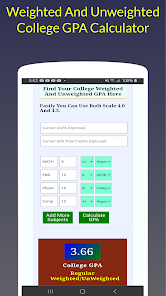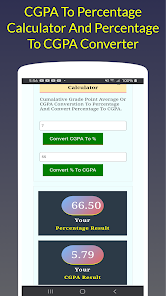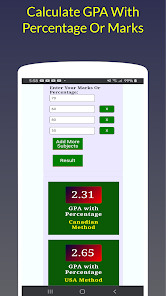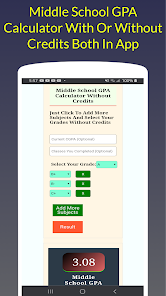# College GPA Calculator + High School GPA + CGPA

500+Everyone----------------------------------------------------------------------------------------------------------------------
A+ 90-100 4.3
A 85-89 4.0
A- 80-84 3.7
B+ 77-79 3.3
B 73-76 3.0
B- 70-72 2.7
C+ 65-69 2.3
C 60-64 2.0
C- 55-59 1.7
D 50-54 1.0
F 0-49 0.0
------------------------------------------------------------------------------------------------
College GPA | High School GPA | Middle School GPA Formula
-----------------------------------------------------------------------------------------------
Credit Hours 1 - 5 x Grade Value B+=3.3
Credit Hours 2 - 5 x Grade Value C=2.0
5 x 3.3 = 16.5
5 x 2.0 = 10.0
16.5 + 10.0 = 26.5
5 + 5 = 10
26.5 / 10 = 2.65
--------------------------------------------------------------------------------
Convert Percentage to CGPA Formula
Percentage 63.65 / 9.5 = 6.7
---------------------------------------------------------------------------------
CGPA (Cumulative GPA) Formula Or How To Calculate High School Cumulative GPA
--------------------------------------------------------------------------------------------------------
Total All Semester 1 Credits - 25 x Total Semester 1 GPA 3.6
Total All Semester 2 Credits - 23 x Total Semester 2 GPA 3.0
25 x 3.6 = 90
23 x 3.0 = 69
25 + 23 = 48
90 + 69 = 159
159 / 48 = 3.31
----------------------------------------------------------------------------------------------
Updated on
Apr 8, 2021

## Data safety